# How to use COUNT function in Microsoft Excel

In this article, we’ll learn how to use COUNT function in Microsoft Excel.

In Excel COUNT Function returns the number of cells that contain numerical values, including numbers and dates from a set of data. We can either supply values directly to the function or as a cell reference to the location in the worksheet.

Let’s take examples and understand:

We have data in which we have taken 5 examples: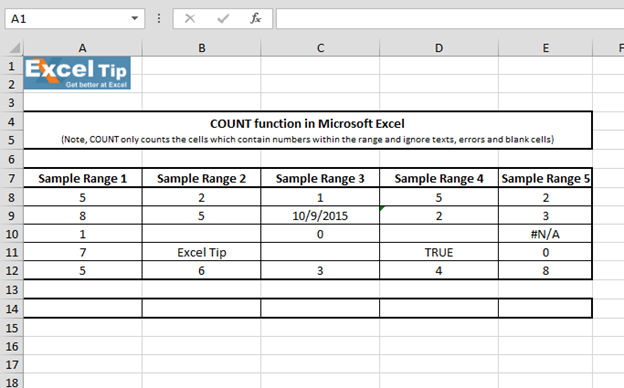1st Example:

In the 1st example, we will enter function in cell A14 by selecting the range A8:A12. Then we’ll see how COUNT Function performs.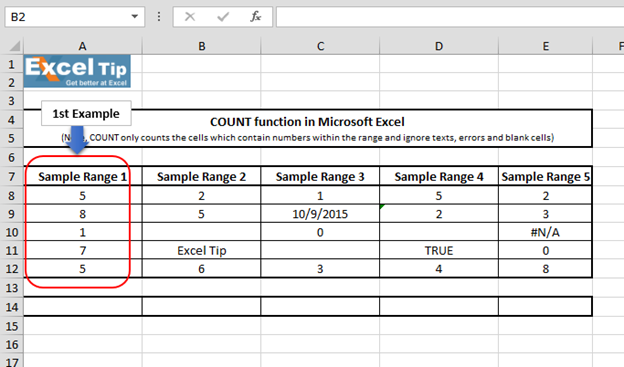• Enter the function in cell A14
• =COUNT(A8:A12)
• Press Enter
• The function returns 5, because range A8:A12 contains numerical values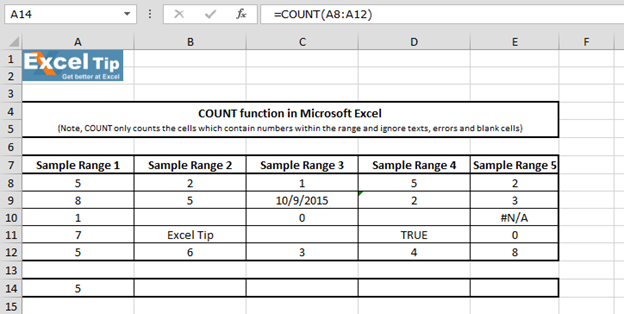2ndExample:

In this example, range contains text cell and blank cell.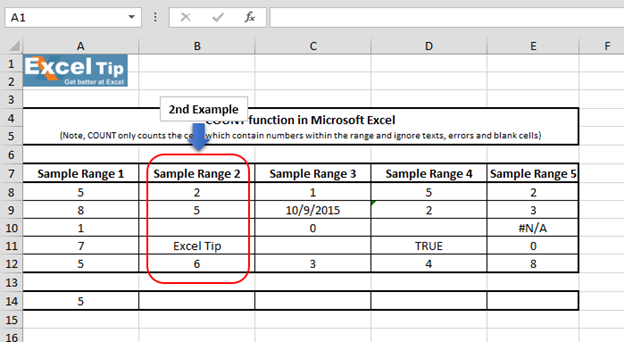• Enter the function in cell B14
• =COUNT(B8:B12)
• Press Enter
• The function returns 3, whereas COUNT function does not include blank cells, text or cells which cannot be translated into numbers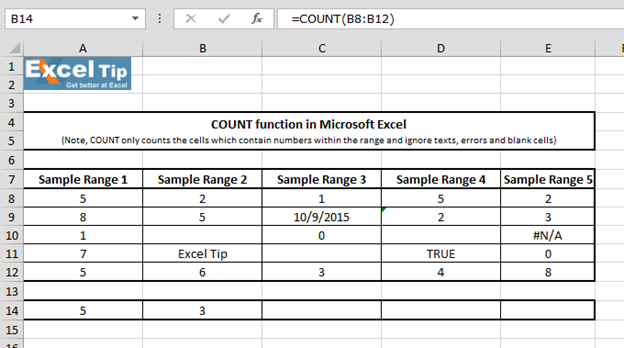3rdExample:

In this example, we’ll see how COUNT function will work when the range has date.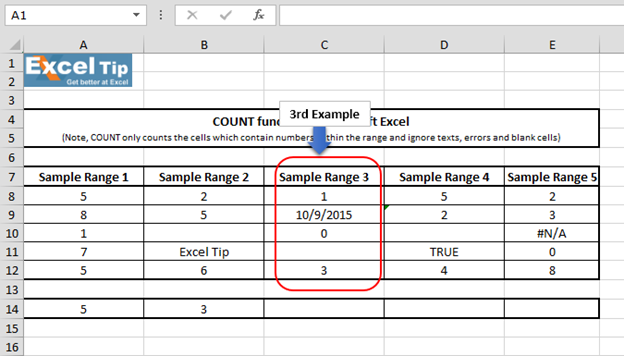• Enter the function in cell C14
• =COUNT(C8:C12)
• Press Enter
• The function will return 4 even when we have date in the range. That’s because Excel reads date as number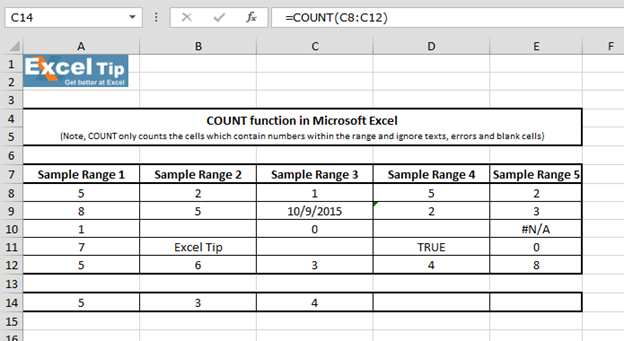4th Example:

In this example, we have range which contains blank cell, text and a number which is stored as text.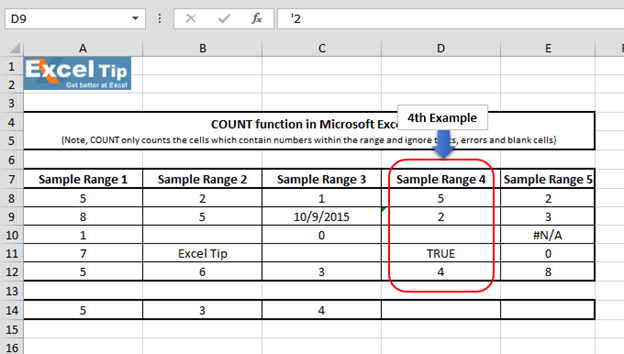• Enter the function in cell D14
• =COUNT(D8:D12)
• Press Enter
• The function returns 2 because COUNTA function ignores other text and prefers numbers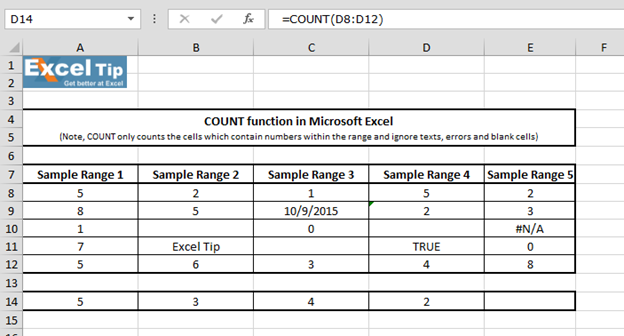5th Example:

In this example, we have taken #N/A error value within range for which we want to enter the formula.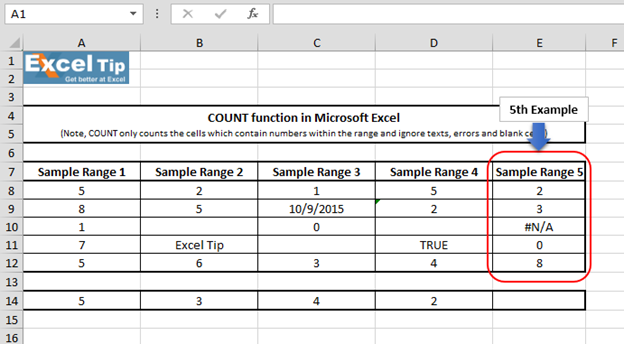• Enter the function in cell E14
• =COUNT(E8:E12)
• Press Enter
• The function returns 4 --it is strange, but it is because function does not count the cell which has error value in it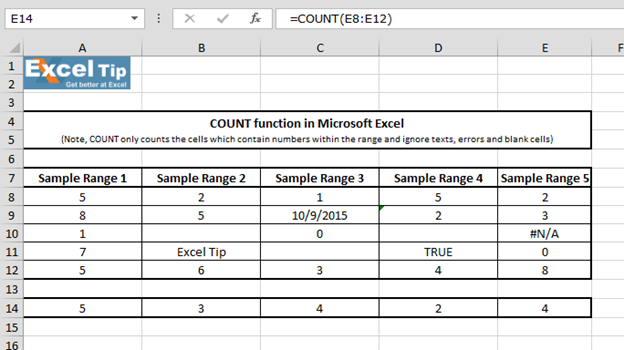In this way we can use COUNT function in Microsoft Excel.# Video: How to use COUNT function in Microsoft Excel

Watch the steps in this short video, and the written instructions are above the video